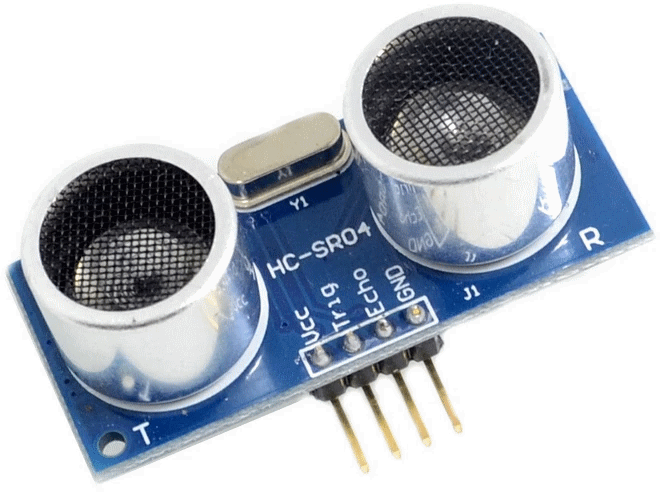components:hc-sr04

# HC-SR04 - Ultrasonic Distance Measure

Ultrasonic Distance Measuring SensorModule main technical parameters

• Working Voltage : 3.3 - 5V(DC)
• Static current: Less than 2mA.
• Output signal: Electric frequency signal, high level 5V, low level 0V.
• Sensor angle: Not more than 15 degrees.
• Detection distance: 2cm-450cm.
• High precision: Up to 0.3cm
• Input trigger signal: 10us TTL impulse
• Echo signal : output TTL PWL signal

Module Working Principle

• Adopt IO trigger through supplying at least 10us sequence of high level signal.
• The module automatically send eight 40khz square wave and automatically detect whether receive the returning pulse signal.
• If there is signals returning, through outputting high level and the time of high level continuing is the time of that from the ultrasonic transmitting to receiving.
`Test distance =  (high level time * sound velocity (340M/S) / 2.`

# Code

```from HCSR04 import HCSR04
import time

sensor = HCSR04(trigger_pin=12, echo_pin=14, echo_timeout_us=10000)
while True:
distance = sensor.distance_cm()
print('Distance:', distance, 'cm')
time.sleep(0.1)```
HCSR04.py
```import machine, time
from machine import Pin

__version__ = '0.2.0'
__author__ = 'Roberto Sánchez'

class HCSR04:
"""
Driver to use the untrasonic sensor HC-SR04.
The sensor range is between 2cm and 4m.
The timeouts received listening to echo pin are converted to OSError('Out of range')
"""
# echo_timeout_us is based in chip range limit (400cm)
def __init__(self, trigger_pin, echo_pin, echo_timeout_us=500*2*30):
"""
trigger_pin: Output pin to send pulses
echo_pin: Readonly pin to measure the distance. The pin should be protected with 1k resistor
echo_timeout_us: Timeout in microseconds to listen to echo pin.
By default is based in sensor limit range (4m)
"""
self.echo_timeout_us = echo_timeout_us
# Init trigger pin (out)
self.trigger = Pin(trigger_pin, mode=Pin.OUT, pull=None)
self.trigger.value(0)

# Init echo pin (in)
self.echo = Pin(echo_pin, mode=Pin.IN, pull=None)

def _send_pulse_and_wait(self):
"""
Send the pulse to trigger and listen on echo pin.
We use the method `machine.time_pulse_us()` to get the microseconds until the echo is received.
"""
self.trigger.value(0) # Stabilize the sensor
time.sleep_us(5)
self.trigger.value(1)
# Send a 10us pulse.
time.sleep_us(10)
self.trigger.value(0)
try:
pulse_time = machine.time_pulse_us(self.echo, 1, self.echo_timeout_us)
return pulse_time
except OSError as ex:
if ex.args == 110: # 110 = ETIMEDOUT
raise OSError('Out of range')
raise ex

def distance_mm(self):
"""
Get the distance in milimeters without floating point operations.
"""
pulse_time = self._send_pulse_and_wait()

# To calculate the distance we get the pulse_time and divide it by 2
# (the pulse walk the distance twice) and by 29.1 becasue
# the sound speed on air (343.2 m/s), that It's equivalent to
# 0.34320 mm/us that is 1mm each 2.91us
# pulse_time // 2 // 2.91 -> pulse_time // 5.82 -> pulse_time * 100 // 582
mm = pulse_time * 100 // 582
return mm

def distance_cm(self):
"""
Get the distance in centimeters with floating point operations.
It returns a float
"""
pulse_time = self._send_pulse_and_wait()

# To calculate the distance we get the pulse_time and divide it by 2
# (the pulse walk the distance twice) and by 29.1 becasue
# the sound speed on air (343.2 m/s), that It's equivalent to
# 0.034320 cm/us that is 1cm each 29.1us
cms = (pulse_time / 2) / 29.1
return cms```
• components/hc-sr04.txt